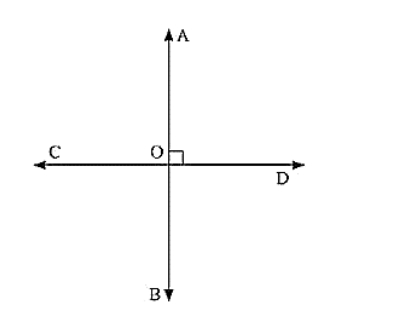# If one of the four angles formed by two intersecting lines is a right angle.

Question:

If one of the four angles formed by two intersecting lines is a right angle. Then show that each of the four angles is a right angle.Solution:

Given,

AB and CD are two lines intersecting at O, such that

∠BOC = 90, ∠AOC = 90 ∠AOD = 90° and ∠BOD = 90

Proof:

Given that B0C = 90

Vertically opposite angles are equal

BOC = AOD = 90

A0C, BOC are a Linear pair of angles

∠AOC + ∠BOC = 180°

[Linear pair]

AOC + 90 = 180

AOC = 90

Vertically opposite angles

Therefore, AOC = BOD = 90

Hence, AOC = BOC = BOD = AOD = 90